This is an example of Number of the Week using 461

Print out or copy the page PDF or WordUse words to “WRITE” your number–four hundred sixty one

Use pictures to “DRAW” your number

• use a box for 1000, a square for 100, a line for 10 and a dot for 1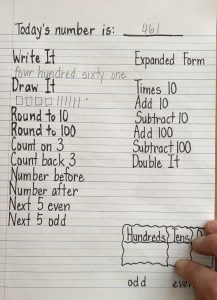Round to the nearest 10 and 100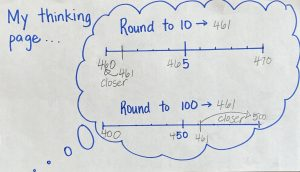• 461 is closer to 460
• 461 is closer to 500

The next few sections are related to counting

• Count on 3 and count back 3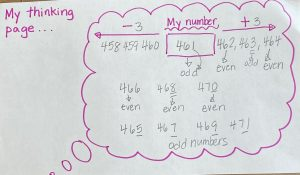• Number before and number after are completed because you already counted on
• Next 5 even and next 5 odd
• remember odd numbers end with 1, 3, 5, 7, 9
• remember even numbers end with 0, 2, 4, 6, 8

Expanded form and the place value chart are also related

• There are 4 hundreds
• There are 6 tens
• There are 1 ones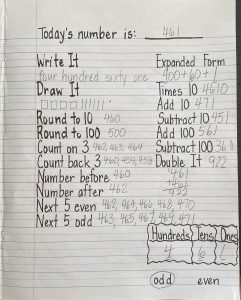Times 10

• When you multiply by 10, you add a 0 at the end of a number
• For example 10 groups of 3 is 3+3+3+3+3+3+3+3+3+3=30 or 3×10=30
• We have also practiced counting by 10s and the numbers always end in 0

The next section is also related–you can use scrap paper for this section to help you out

• Add 10 and subtract 10
• 461+10=471
• 461-10=451
• Add 100 and subtract 100
• 461+100=561
• 461-100=361

Double it

• 461=461=922–CAREFUL WHEN YOU ADD, YOU HAVE TO CARRY

Now, is 461 odd or even?  We went through this already–it ends with a 1 so, it is ODD!

Extension activities

We will continue to use 461 as an example:

1. Use this number and create a story problem

• There are 461 trees in the forest.  300 are Douglas Fir trees, 100 are Hemlock trees, 30 are Yew trees. How many are Cedar trees?
• 300+100+30=430
• 461-430=31  There are 31 Cedar trees in the forest.

2. Find objects or items around the house to estimate these many things

• A new package of paper has around 461 sheets of paper

3. Draw a picture of this number using cents as many different ways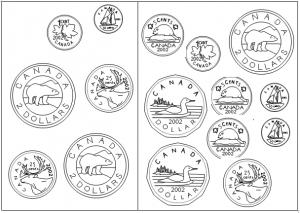4. Draw a picture of this number using different base ten block symbols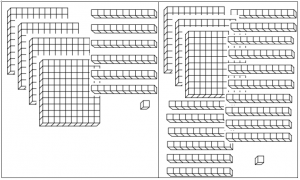5. Create your own symbols to count this number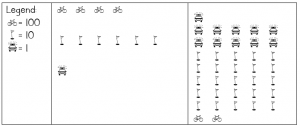6. Hunt for this number inside your home, safely outside or in a story you have read or heard and draw a picture with labels–hint–sometimes, these numbers are from our read alouds

Remember to try your best with your number and if you have questions, let me know.